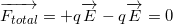# Torque on a Dipole in a uniform Electric field | cbse24.com

### Torque on a dipole in a uniform electric field:

As shown in fig, consider an electric dipole consisting of charge +q and -Q and of length 2a placed in a uniform electric field E making an angle θ with it. It has a dipole moment of magnitude.

P=q x 2aTorque on a dipole in a uniform electric field

Force exerted on charge +q by field  E⃗ =+q.E      (along with E )

Force exerted on the charge -q by field  E⃗ =qE     (opposite to E)Simulation of Torque on a dipole in a uniform electric field

Hence the net translating force on a dipole in a uniform electric field is zero. But the two equal and opposite forces act at different points of the dipole. They form a couple that exerts a torque.

Torque = Either force x Perpendicular distance between the two forces

τ=qE×2a.sinθ=(q×2a)E.sinθ

Where (p=q x 2a)

τ=pEsinθThe direction of torque as given by right-hand screw rule

As the direction of toque  τ is perpendicular to both p and E, so we can write
τ=p×E

When the dipole is released , the torque tends to align the dipole with the field E i.e. , tends to reduce angle θ to 0.When the dipole gets aligned with E the torque becomes zero.

τ=0  ( torque align with E)

Clearly , the torque on the dipole will be maximum when the dipole is held perpendicular to E. Thus

τmax  =p.Esin90=p.E

### Dipole moment:

τ=pEsinθ

If  E=1 unit, θ=90    τ = p

Hence dipole moment may be defined as the torque acting on an electric dipole , placed perpendicular to a uniform electric field of unit strength.

*